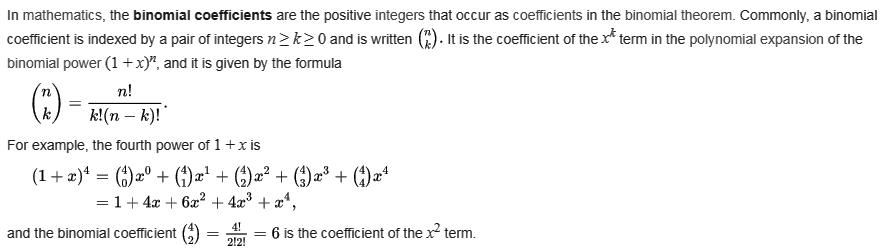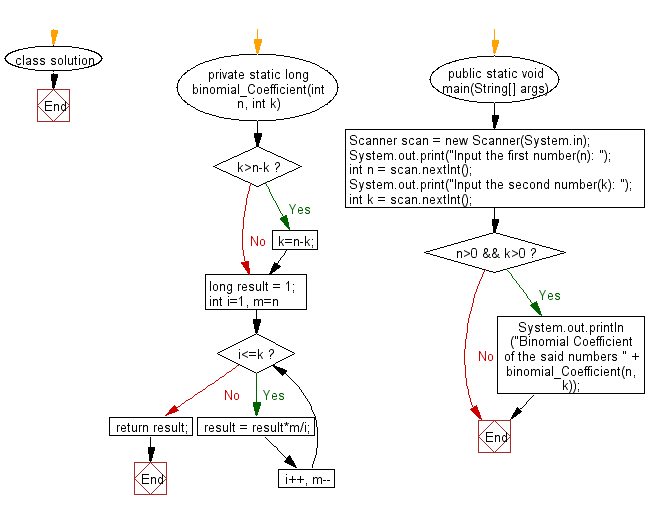﻿ Java Math Exercises: Calculate the Binomial Coefficient of two positive numbers - w3resource# Java Math Exercises: Calculate the Binomial Coefficient of two positive numbers

## Java Math Exercises: Exercise-24 with Solution

From Wikipedia,Write a Java program to calculate the Binomial Coefficient of two positive numbers.

Sample Solution:

Java Code:

``````import java.util.*;

class solution {

private static long binomial_Coefficient(int n, int k)
{
if (k>n-k)
k=n-k;

long result = 1;
for (int i=1, m=n; i<=k; i++, m--)
result = result*m/i;
return result;
}

public static void main(String[] args)
{
Scanner scan = new Scanner(System.in);
System.out.print("Input the first number(n): ");
int n = scan.nextInt();
System.out.print("Input the second number(k): ");
int k = scan.nextInt();
if (n>0 && k>0)
{
System.out.println("Binomial Coefficient of the said numbers " + binomial_Coefficient(n, k));
}
}
}
``````

Sample Output:

```Input the first number(n):  10
Input the second number(k):  2
Binomial Coefficient of the said numbers 45
```

Flowchart:Java Code Editor:

What is the difficulty level of this exercise?

﻿

## Java: Tips of the Day

Parsing dates:

```import java.io.*;
import java.util.*;
import java.text.*;

String s = "2001/09/23 14:39";

SimpleDateFormat formatter = new SimpleDateFormat ("yyyy/MM/dd H:mm");
Date d = formatter.parse(s, new ParsePosition(0));
```